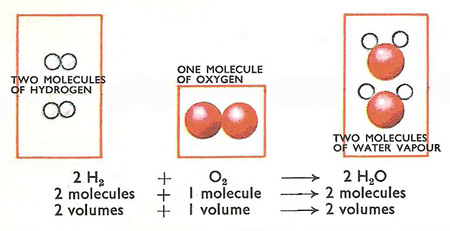# Question #5aa17

May 13, 2017

The chemical reaction is based from the number of moles of each species participating in the reaction and not by its volume.

#### Explanation:

This is how it works:

First, this is the reaction for the formation of water:
${H}_{2} + \frac{1}{2} {O}_{2} \rightarrow {H}_{2} O$

All chemical reactions are based on the number of moles (see those coefficients?) and not in their volume. Meaning 1 mole of ${H}_{2}$(hydrogen gas) will react with a half mole of ${O}_{2}$(oxygen gas) to form 1 mole of liquid water.

Jun 7, 2017

#### Explanation:

Avogadro's Hypothesis states that equal volumes of gases at the same temperature and pressure contain equal numbers of molecules.

The chemical equation for the formation of water is

$\text{2H"_2"(g)" + "O"_2"(g)" → 2"H"_2"O(g)}$We could also write

$\text{2 mL + 1 mL → 2 mL}$

or

$\text{100 mL + 50 mL → 100 mL}$

The volumes will always be in the same $2 : 1 : 2$ ratio as the coefficients in the balanced chemical equation.